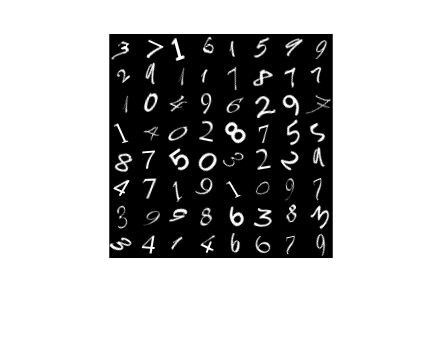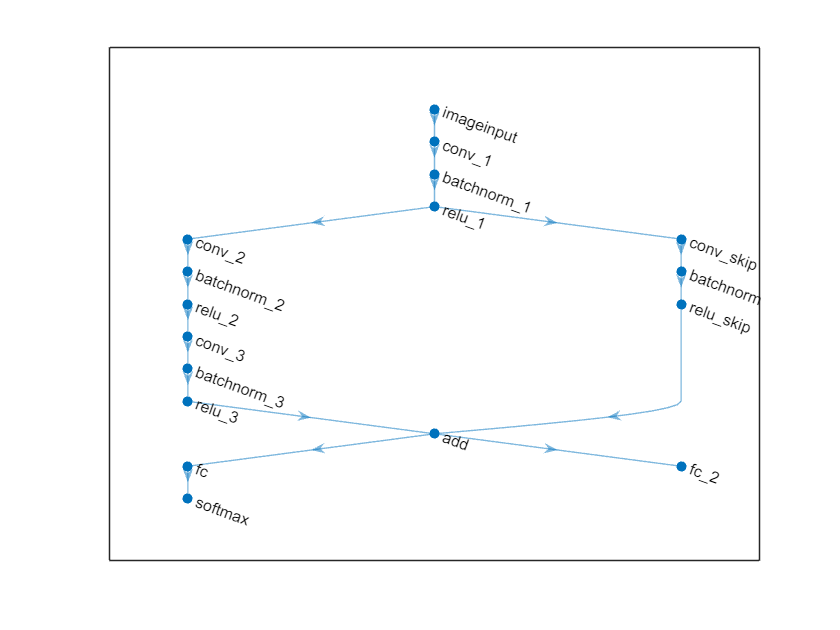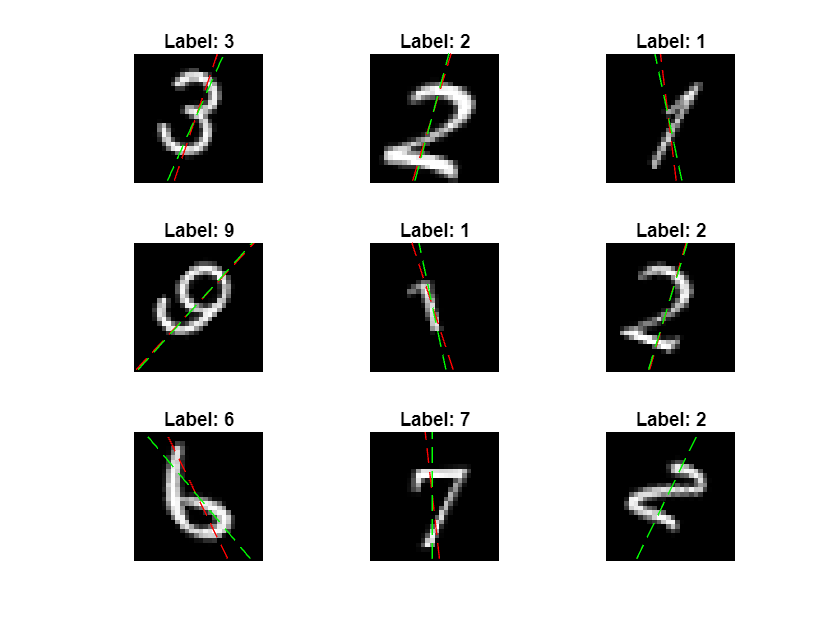## Train Network with Multiple Outputs

This example shows how to train a deep learning network with multiple outputs that predict both labels and angles of rotations of handwritten digits.

To train a network with multiple outputs, you must specify the network as a function and train it using a custom training loop.

The `digitTrain4DArrayData` function loads the images, their digit labels, and their angles of rotation from the vertical.

```[XTrain,YTrain,anglesTrain] = digitTrain4DArrayData; classNames = categories(YTrain); numClasses = numel(classNames); numObservations = numel(YTrain);```

View some images from the training data.

```idx = randperm(numObservations,64); I = imtile(XTrain(:,:,:,idx)); figure imshow(I)```### Define Deep Learning Model

Define the following network that predicts both labels and angles of rotation.

• A convolution-batchnorm-ReLU block with 16 5-by-5 filters.

• A branch of two convolution-batchnorm blocks each with 32 3-by-3 filters with a ReLU operation between

• A skip connection with a convolution-batchnorm block with 32 1-by-1 convolutions.

• Combine both branches using addition followed by a ReLU operation

• For the regression output, a branch with a fully connected operation of size 1 (the number of responses).

• For classification output, a branch with a fully connected operation of size 10 (the number of classes) and a softmax operation.#### Define and Initialize Model Parameters and State

Define the parameters for each of the operations and include them in a struct. Use the format `parameters.OperationName.ParameterName` where `parameters` is the struct, O`perationName` is the name of the operation (for example "conv_1") and `ParameterName` is the name of the parameter (for example, "Weights").

Create a struct `parameters` containing the model parameters. Initialize the learnable layer weights using the example function `initializeGaussian`, listed at the end of the example. Initialize the learnable layer biases with zeros. Initialize the batch normalization offset and scale parameters with zeros and ones, respectively.

To perform training and inference using batch normalization layers, you must also manage the network state. Before prediction, you must specify the dataset mean and variance derived from the training data. Create a struct `state` containing the state parameters. Initialize the batch normalization trained mean and trained variance states with zeros and ones, respectively.

```parameters.conv1.Weights = dlarray(initializeGaussian([5,5,1,16])); parameters.conv1.Bias = dlarray(zeros(16,1,'single')); parameters.batchnorm1.Offset = dlarray(zeros(16,1,'single')); parameters.batchnorm1.Scale = dlarray(ones(16,1,'single')); state.batchnorm1.TrainedMean = zeros(16,1,'single'); state.batchnorm1.TrainedVariance = ones(16,1,'single'); parameters.convSkip.Weights = dlarray(initializeGaussian([1,1,16,32])); parameters.convSkip.Bias = dlarray(zeros(32,1,'single')); parameters.batchnormSkip.Offset = dlarray(zeros(32,1,'single')); parameters.batchnormSkip.Scale = dlarray(ones(32,1,'single')); state.batchnormSkip.TrainedMean = zeros(32,1,'single'); state.batchnormSkip.TrainedVariance = ones(32,1,'single'); parameters.conv2.Weights = dlarray(initializeGaussian([3,3,16,32])); parameters.conv2.Bias = dlarray(zeros(32,1,'single')); parameters.batchnorm2.Offset = dlarray(zeros(32,1,'single')); parameters.batchnorm2.Scale = dlarray(ones(32,1,'single')); state.batchnorm2.TrainedMean = zeros(32,1,'single'); state.batchnorm2.TrainedVariance = ones(32,1,'single'); parameters.conv3.Weights = dlarray(initializeGaussian([3,3,32,32])); parameters.conv3.Bias = dlarray(zeros(32,1,'single')); parameters.batchnorm3.Offset = dlarray(zeros(32,1,'single')); parameters.batchnorm3.Scale = dlarray(ones(32,1,'single')); state.batchnorm3.TrainedMean = zeros(32,1,'single'); state.batchnorm3.TrainedVariance = ones(32,1,'single'); parameters.fc2.Weights = dlarray(initializeGaussian([10,6272])); parameters.fc2.Bias = dlarray(zeros(numClasses,1,'single')); parameters.fc1.Weights = dlarray(initializeGaussian([1,6272])); parameters.fc1.Bias = dlarray(zeros(1,1,'single'));```

View the struct of the parameters.

`parameters`
```parameters = struct with fields: conv1: [1×1 struct] batchnorm1: [1×1 struct] convSkip: [1×1 struct] batchnormSkip: [1×1 struct] conv2: [1×1 struct] batchnorm2: [1×1 struct] conv3: [1×1 struct] batchnorm3: [1×1 struct] fc2: [1×1 struct] fc1: [1×1 struct] ```

View the parameters for the "conv1" operation.

`parameters.conv1`
```ans = struct with fields: Weights: [5×5×1×16 dlarray] Bias: [16×1 dlarray] ```

View the struct of the state.

`state`
```state = struct with fields: batchnorm1: [1×1 struct] batchnormSkip: [1×1 struct] batchnorm2: [1×1 struct] batchnorm3: [1×1 struct] ```

View the state parameters for the "batchnorm1" operation.

`state.batchnorm1`
```ans = struct with fields: TrainedMean: [16×1 single] TrainedVariance: [16×1 single] ```

#### Define Model Function

Create the function `model`, listed at the end of the example, that computes the outputs of the deep learning model described earlier.

The function `model` takes the input data `dlX`, the model parameters `parameters`, the flag `doTraining` which specifies whether to model should return outputs for training or prediction, and the network state `state`. The network outputs the predictions for the labels, the predictions for the angles, and the updated network state.

Create the function `modelGradients`, listed at the end of the example, that takes a mini-batch of input data `dlX` with corresponding targets `T1` and `T2` containing the labels and angles, respectively, and returns the gradients of the loss with respect to the learnable parameters, the updated network state, and the corresponding loss.

### Specify Training Options

Specify the training options.

```learnRate = 0.001; momentum = 0.9; numEpochs = 30; miniBatchSize = 128; plots = "training-progress"; trailingAvg = []; trailingAvgSq = []; numIterationsPerEpoch = floor(numObservations./miniBatchSize);```

Train on a GPU if one is available. This requires Parallel Computing Toolbox™. Using a GPU requires Parallel Computing Toolbox™ and a CUDA® enabled NVIDIA® GPU with compute capability 3.0 or higher.

`executionEnvironment = "auto";`

### Train Model

Train the model using a custom training loop.

For each epoch, shuffle the data and loop over mini-batches of data. At the end of each epoch, display the training progress.

For each mini-batch:

• Convert the labels to dummy variables.

• Convert the data to `dlarray` objects with underlying type single and specify the dimension labels `'SSCB'` (spatial, spatial, channel, batch).

• For GPU training, convert to `gpuArray` objects.

• Evaluate the model gradients and loss using `dlfeval` and the `modelGradients` function.

• Update the network parameters using the `adamupdate` function.

Initialize the training progress plot.

```if plots == "training-progress" figure lineLossTrain = animatedline; xlabel("Iteration") ylabel("Loss") end```

Train the model.

```iteration = 0; start = tic; % Loop over epochs. for epoch = 1:numEpochs % Shuffle data. idx = randperm(numObservations); XTrain = XTrain(:,:,:,idx); YTrain = YTrain(idx); anglesTrain = anglesTrain(idx); % Loop over mini-batches for i = 1:numIterationsPerEpoch iteration = iteration + 1; idx = (i-1)*miniBatchSize+1:i*miniBatchSize; % Read mini-batch of data and convert the labels to dummy % variables. X = XTrain(:,:,:,idx); Y1 = zeros(numClasses, miniBatchSize, 'single'); for c = 1:numClasses Y1(c,YTrain(idx)==classNames(c)) = 1; end Y2 = anglesTrain(idx)'; Y2 = single(Y2); % Convert mini-batch of data to dlarray. dlX = dlarray(X,'SSCB'); % If training on a GPU, then convert data to gpuArray. if (executionEnvironment == "auto" && canUseGPU) || executionEnvironment == "gpu" dlX = gpuArray(dlX); end % Evaluate the model gradients, state, and loss using dlfeval and the % modelGradients function. [gradients,state,loss] = dlfeval(@modelGradients, dlX, Y1, Y2, parameters, state); % Update the network parameters using the Adam optimizer. [parameters,trailingAvg,trailingAvgSq] = adamupdate(parameters,gradients, ... trailingAvg,trailingAvgSq,iteration); % Display the training progress. if plots == "training-progress" D = duration(0,0,toc(start),'Format','hh:mm:ss'); addpoints(lineLossTrain,iteration,double(gather(extractdata(loss)))) title("Epoch: " + epoch + ", Elapsed: " + string(D)) drawnow end end end```### Test Model

Test the classification accuracy of the model by comparing the predictions on a test set with the true labels and angles

`[XTest,YTest,anglesTest] = digitTest4DArrayData;`

Convert the data to a `dlarray` object with dimension format `'SSCB'`. For GPU prediction, also convert the data to `gpuArray`.

```dlXTest = dlarray(XTest,'SSCB'); if (executionEnvironment == "auto" && canUseGPU) || executionEnvironment == "gpu" dlXTest = gpuArray(dlXTest); end```

To predict the labels and angles of the validation data, use the model function with the `doTraining` option set to `false`.

```doTraining = false; [dlYPred,anglesPred] = model(dlXTest, parameters,doTraining,state);```

Evaluate the classification accuracy.

```[~,idx] = max(extractdata(dlYPred),[],1); labelsPred = classNames(idx); accuracy = mean(labelsPred==YTest)```
```accuracy = 0.9892 ```

Evaluate the regression accuracy.

`angleRMSE = sqrt(mean((extractdata(anglesPred) - anglesTest').^2))`
```angleRMSE = 1×1 single gpuArray 7.5851 ```

View some of the images with their predictions. Display the predicted angles in red and the correct labels in green.

```idx = randperm(size(XTest,4),9); figure for i = 1:9 subplot(3,3,i) I = XTest(:,:,:,idx(i)); imshow(I) hold on sz = size(I,1); offset = sz/2; thetaPred = extractdata(anglesPred(idx(i))); plot(offset*[1-tand(thetaPred) 1+tand(thetaPred)],[sz 0],'r--') thetaValidation = anglesTest(idx(i)); plot(offset*[1-tand(thetaValidation) 1+tand(thetaValidation)],[sz 0],'g--') hold off label = string(labelsPred(idx(i))); title("Label: " + label) end```### Model Function

The function `model` takes the input data `dlX`, the model parameters `parameters`, the flag `doTraining` which specifies whether to model should return outputs for training or prediction, and the network state `state`. The network outputs the predictions for the labels, the predictions for the angles, and the updated network state.

```function [dlY1,dlY2,state] = model(dlX,parameters,doTraining,state) % Convolution W = parameters.conv1.Weights; B = parameters.conv1.Bias; dlY = dlconv(dlX,W,B,'Padding',2); % Batch normalization, ReLU Offset = parameters.batchnorm1.Offset; Scale = parameters.batchnorm1.Scale; trainedMean = state.batchnorm1.TrainedMean; trainedVariance = state.batchnorm1.TrainedVariance; if doTraining [dlY,trainedMean,trainedVariance] = batchnorm(dlY,Offset,Scale,trainedMean,trainedVariance); % Update state state.batchnorm1.TrainedMean = trainedMean; state.batchnorm1.TrainedVariance = trainedVariance; else dlY = batchnorm(dlY,Offset,Scale,trainedMean,trainedVariance); end dlY = relu(dlY); % Convolution, batch normalization (Skip connection) W = parameters.convSkip.Weights; B = parameters.convSkip.Bias; dlYSkip = dlconv(dlY,W,B,'Stride',2); Offset = parameters.batchnormSkip.Offset; Scale = parameters.batchnormSkip.Scale; trainedMean = state.batchnormSkip.TrainedMean; trainedVariance = state.batchnormSkip.TrainedVariance; if doTraining [dlYSkip,trainedMean,trainedVariance] = batchnorm(dlYSkip,Offset,Scale,trainedMean,trainedVariance); % Update state state.batchnormSkip.TrainedMean = trainedMean; state.batchnormSkip.TrainedVariance = trainedVariance; else dlYSkip = batchnorm(dlYSkip,Offset,Scale,trainedMean,trainedVariance); end % Convolution W = parameters.conv2.Weights; B = parameters.conv2.Bias; dlY = dlconv(dlY,W,B,'Padding',1,'Stride',2); % Batch normalization, ReLU Offset = parameters.batchnorm2.Offset; Scale = parameters.batchnorm2.Scale; trainedMean = state.batchnorm2.TrainedMean; trainedVariance = state.batchnorm2.TrainedVariance; if doTraining [dlY,trainedMean,trainedVariance] = batchnorm(dlY,Offset,Scale,trainedMean,trainedVariance); % Update state state.batchnorm2.TrainedMean = trainedMean; state.batchnorm2.TrainedVariance = trainedVariance; else dlY = batchnorm(dlY,Offset,Scale,trainedMean,trainedVariance); end dlY = relu(dlY); % Convolution W = parameters.conv3.Weights; B = parameters.conv3.Bias; dlY = dlconv(dlY,W,B,'Padding',1); % Batch normalization Offset = parameters.batchnorm3.Offset; Scale = parameters.batchnorm3.Scale; trainedMean = state.batchnorm3.TrainedMean; trainedVariance = state.batchnorm3.TrainedVariance; if doTraining [dlY,trainedMean,trainedVariance] = batchnorm(dlY,Offset,Scale,trainedMean,trainedVariance); % Update state state.batchnorm3.TrainedMean = trainedMean; state.batchnorm3.TrainedVariance = trainedVariance; else dlY = batchnorm(dlY,Offset,Scale,trainedMean,trainedVariance); end % Addition, ReLU dlY = dlYSkip + dlY; dlY = relu(dlY); % Fully connect (angles) W = parameters.fc1.Weights; B = parameters.fc1.Bias; dlY2 = fullyconnect(dlY,W,B); % Fully connect, softmax (labels) W = parameters.fc2.Weights; B = parameters.fc2.Bias; dlY1 = fullyconnect(dlY,W,B); dlY1 = softmax(dlY1); end```

The `modelGradients` function, takes a mini-batch of input data `dlX` with corresponding targets `T1` and `T2` containing the labels and angles, respectively, and returns the gradients of the loss with respect to the learnable parameters, the updated network state, and the corresponding loss.

```function [gradients,state,loss] = modelGradients(dlX,T1,T2,parameters,state) doTraining = true; [dlY1,dlY2,state] = model(dlX,parameters,doTraining,state); lossLabels = crossentropy(dlY1,T1); lossAngles = mse(dlY2,T2); loss = lossLabels + 0.1*lossAngles; gradients = dlgradient(loss,parameters); end```

### Weights Initialization Function

The `initializeGaussian` function samples weights from a Gaussian distribution with mean 0 and standard deviation 0.01.

```function parameter = initializeGaussian(sz) parameter = randn(sz,'single').*0.01; end```